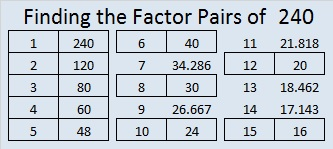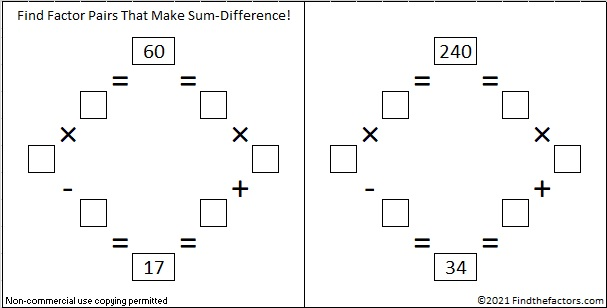# A Forest of 240 Factor Trees

Contents

### Factor Trees for 240:

Because 240 has so many factors, it is possible to make MANY different factor trees that create a forest of 240 factor trees. This post only contains eleven of those many possibilities. The two trees below demonstrate different permutations that can be made from the same basic tree. The mirror images of both, as well as mirror images of parts of either tree, would be other permutations.A good way to make a factor tree for a composite number is to begin with one of its factor pairs and then make factor trees for the composite numbers in that factor pair.

This forest of 240 factor trees is dedicated to Joseph Nebus. Read the comments to his post, You might also like, because, I don’t know why, to discover why I was inspired to create images of parts of this forest.

### Factors of 240:

• 240 is a composite number.
• Prime factorization: 240 = 2 x 2 x 2 x 2 x 3 x 5, which can be written 2⁴ x 3 x 5
• The exponents in the prime factorization are 4, 1 and 1. Adding one to each and multiplying we get (4 + 1)(1 + 1)(1 + 1) = 5 x 2 x 2 = 20. Therefore 240 has 20 factors.
• Factors of 240: 1, 2, 3, 4, 5, 6, 8, 10, 12, 15, 16, 20, 24, 30, 40, 48, 60, 80, 120, 240
• Factor pairs: 240 = 1 x 240, 2 x 120, 3 x 80, 4 x 60, 5 x 48, 6 x 40, 8 x 30, 10 x 24, 12 x 20, or 15 x 16
• Taking the factor pair with the largest square number factor, we get √240 = (√16)(√15) = 4√15 ≈ 15.492### Sum-Difference Puzzle:

60 has six factor pairs. One of those factor pairs adds up to 17, and a different one subtracts to 17. Can you find those factor pairs to solve the first puzzle below?

240 has ten factor pairs. One of them adds up to 34, and another one subtracts to 34. If you can identify those factor pairs, then you can solve the second puzzle.The second puzzle is really just the first puzzle in disguise. Why would I say that?

### Another Fact about the Number 240:

2 + 4 + 6 + 8 + 10 + 12 + 14 + 16 + 18 + 20 + 22 + 24 + 26 + 28 + 30 = 240; that’s the sum of the first 15 even numbers.

## 2 thoughts on “A Forest of 240 Factor Trees”

1.Joseph Nebus

Reblogged this on nebusresearch and commented:
I admit this is a little self-indulgent, but, what the heck. You might also like the factor trees for the extremely factorable number 240 that Ivasallay’s put up at Find The Factors.

This site uses Akismet to reduce spam. Learn how your comment data is processed.